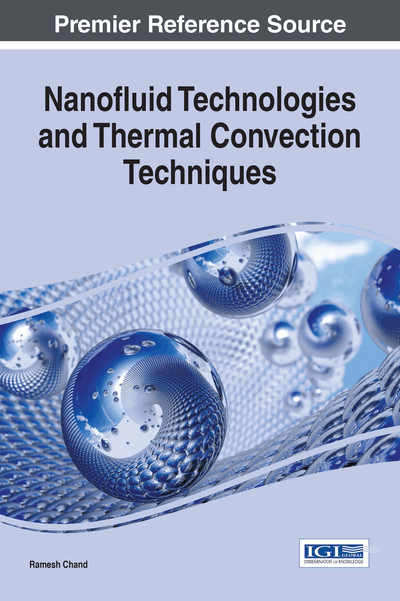# Effect of Variable Gravity on the Thermal Convection in a Layer of Maxwellian Visco-Elastic Nanofluid

Copyright: © 2017 |Pages: 17
DOI: 10.4018/978-1-68318-006-7.ch007
OnDemand PDF Download:
Available
\$37.50
No Current Special Offers

Top

## Mathematical Formulations Of The Problem

### The Physical Problem

In this chapter we shall investigate effect of variable gravity on the onset of thermal convection in a horizontal layer of Maxwellian visco-elastic nanofluid. The physical configuration of the problem to be considered as:

An infinite horizontal layer of Maxwell visco-elastic nanofluid of thickness ‘d’ bounded by horizontal boundaries z = 0 and z = d. Fluid layer is acted upon by a gravity force g(0,0,-g) and and it is assumed that gravity force vector is varies linearly with z i.e., where δh(z) is the variable gravity parameter. Fluid layer is heated from below in such a way that horizontal boundaries z = 0 and z = d respectively maintained at a uniform temperature T0 and T1 (T0 > T1) as shown is Figure 1. The normal component of the nanoparticles flux has to vanish at an impermeable boundaries and the reference scale for temperature and nanoparticles fraction is taken to be T1 and φ0 respectively.

### Assumptions

The mathematical equations describing the physical model are based upon the following assumptions:

• 1.

Thermophysical properties of fluid expect for density in the buoyancy force (Boussinesq Hypothesis) are constant,

• 2.

The fluid phase and nanoparticles are in thermal equilibrium state and thus, the heat flow has been described using one equation model,

• 3.

Dilute mixture,

• 4.

Nanoparticles are spherical,

• 5.

Nanoparticles are non-magnetic,

• 6.

No chemical reactions take place in fluid layer,

• 7.

Negligible viscous dissipation,

• 8.

Radiative heat transfer between the sides of wall is negligible when compared with other modes of the heat transfer,

• 9.

Nanofluid is incompressible, electrically conducting, Newtonian and laminar flow,

• 10.

Each boundary wall is assumed to be impermeable and perfectly thermal conducting.

## Complete Chapter List

Search this Book:
Reset